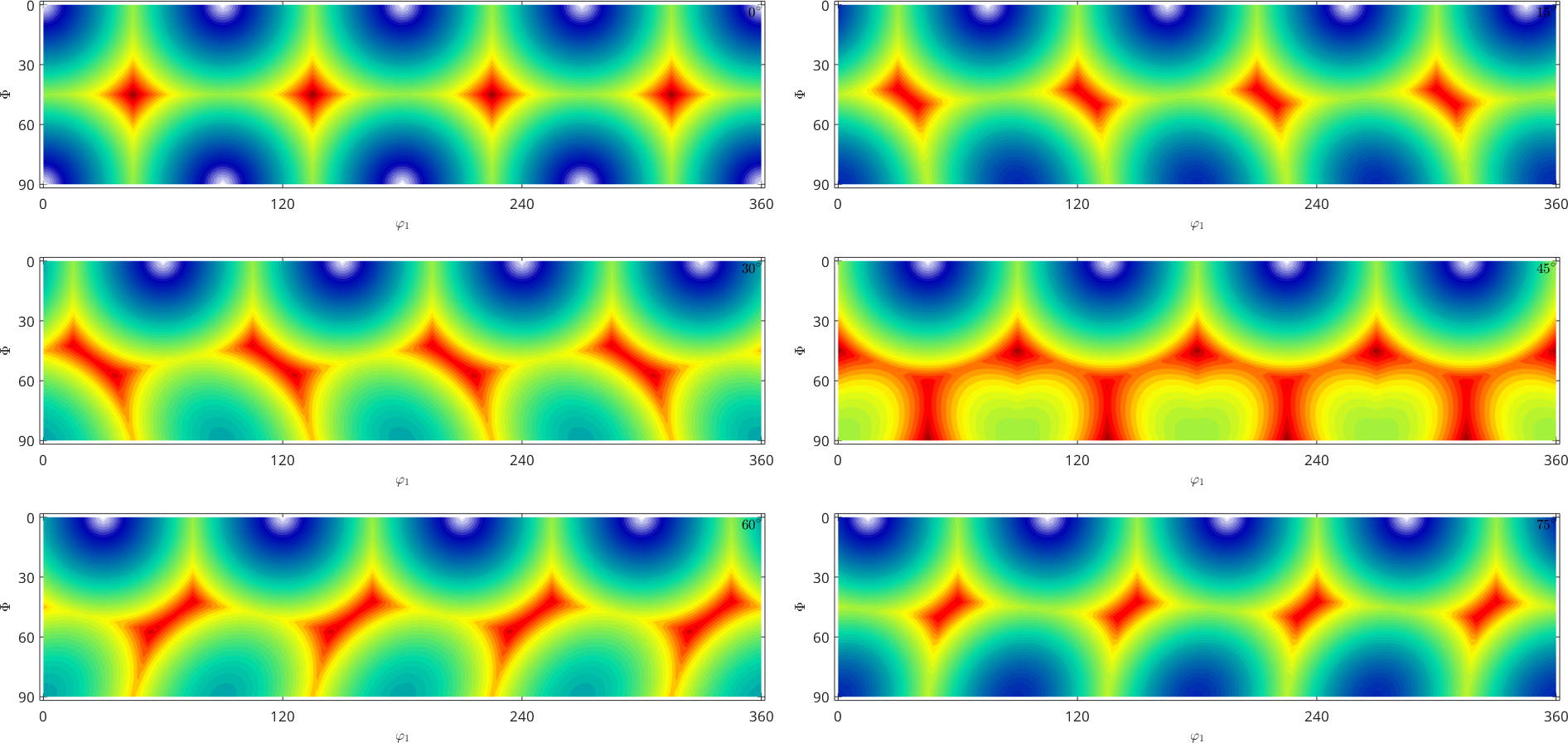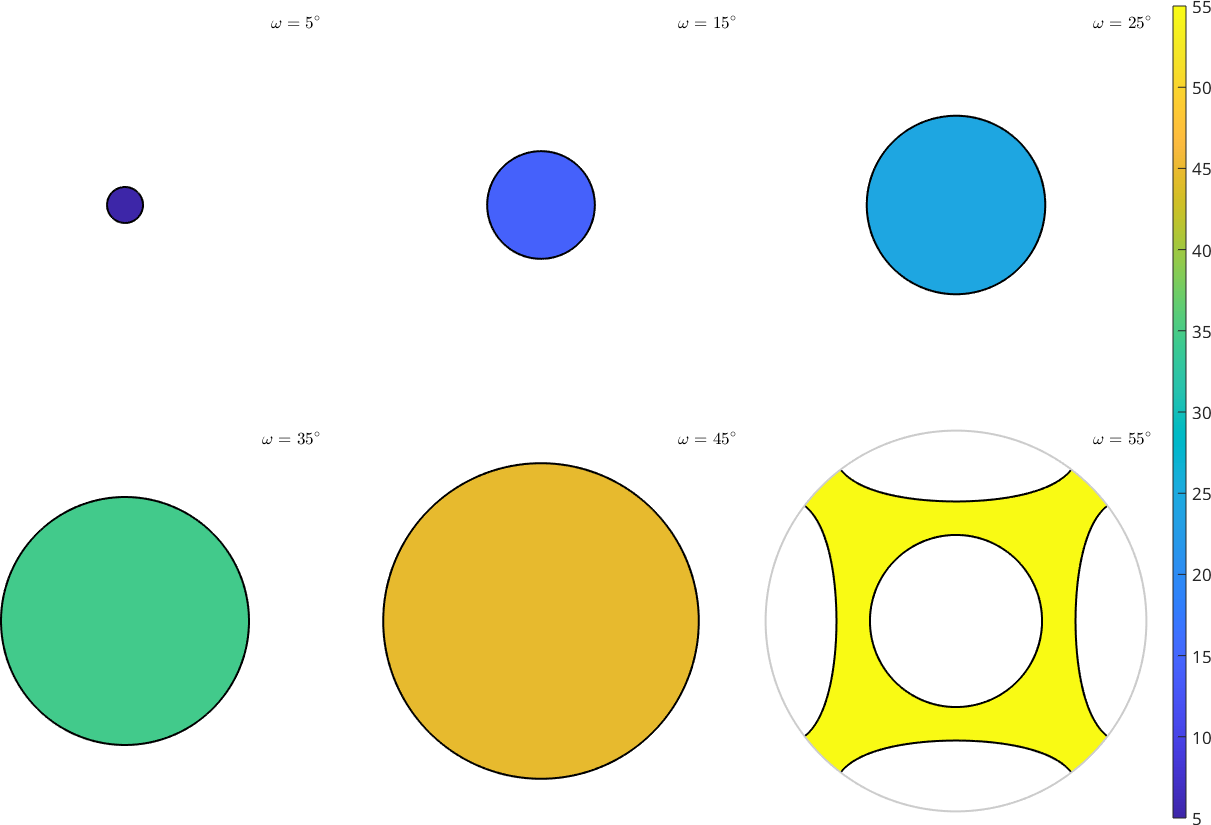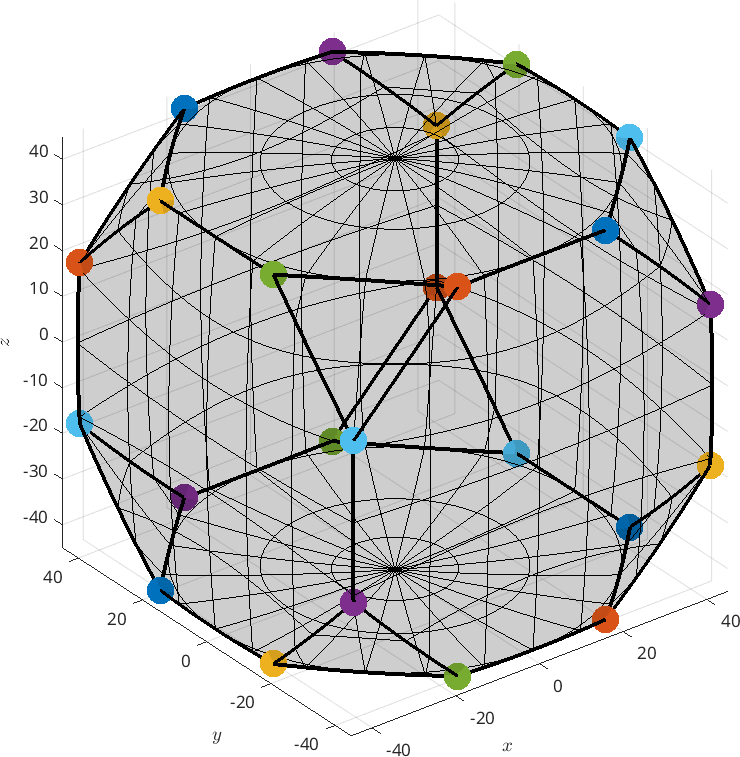Orientation Dependent Functions edit page

An orientation dependent function is a function that assigns to each rotation or orientation a numerical value. An import example of a rotational function is the orientation density function (ODF) that assignes to each crystal orientation the probability of its occurence within a specimen. Other examples are the Schmidt or the Taylor factor as a function of the crystal orientation.

## Definition of a orientation dependent function

Within MTEX a rotational function is represented by a variable of type SO3Fun. Let us consider as an example the function that takes an orientation and returns it rotational angle modulo cubic crystal symmetry. In MTEX the rotational angle is computed by the command angle(ori). In order to turn this correspondence into a SO3Fun we use the command SO3FunHandle and pass the angle command as an anonymous function.

Many more methods for defining orientation dependent functions are discussed here.

The entire information abot the orientation dependent function is now stored in the variable SO3F. In order to determine its value for a specific orientation ori the function eval(ori) is used

## Plotting an orientation Dependent Function

Orientation dependent functions are most of visualized by sections according to the third Euler angle $$\varphi_2$$The plot tells us for which Euler angles the the resulting rotational angle is large and for which Euler angles it is low. The plot of this "angle function" SO3F becomes trivial if represented in an axis angle sectionsas obviously, the function value is constant in each section. Many more methods for visualizing orientation dependent functions are discussed here.

## Computing with orientation dependent functions

The power of representing an orientation dependent functions as a variables of type SO3Fun is that we may apply to it a large number of analysis tools. In particular, one can add, subtract and mutiply orientation dependent functions, plot them in various projections or detect the local minima or maxima. In the case of our example function the local maxima refers to the orientations with maximum rotational angle in cubic symmetry. We may compute them by the command max

We observe that there are exactly six symetrically not equivalent orientations that realize an orientation angle of about 62.994 degree and form the vertices of the fundamental region in orienation space## Representations of Rotational Functions

Internally MTEX represents rotational functions in different ways:

 by a harmonic series expansion as superposition of radial function as superposition of fibre elements as Bingham distribution as sum of different components explicitely given by a formula

All representations allow the same operations which are specified for the abstact class SO3Fun. In particular it is possible to calculate with $$SO(3)$$ functions as with ordinary numbers, i.e., you can add, multiply arbitrary functions, take the mean, integrate them or compute gradients, see Operations.

## Generalizations of Rotational Functions

 rotational vector fields radial rotational functions